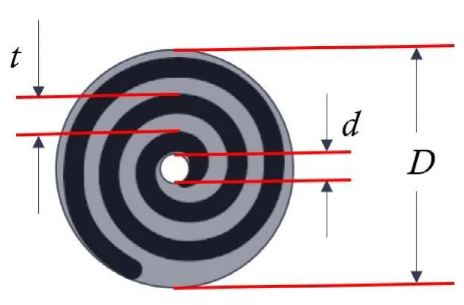# Calculation Method for the Length of Cylindrical Battery ElectrodeThe battery jelly roll is a type of Archimedean spiral. According to the relevant theory, the relationship between the radius r of the jelly roll and the total rotation angle ϕ can be calculated using the following equation：

When the radius of the jelly roll needle in the battery is r0,

Among them, ϕ is the total angle of winding rotation, r0 is the diameter of the inner core winding needle, and the calculation method of spiral parameter a is:

t represents the thickness of the basic unit of the jelly roll. For cylindrical batteries, t is equivalent to the thickness of the positive and negative electrode sheets and the thickness of the two layers of separators, as shown in the diagram.

According to the theory of Archimedean spiral, based on the formula below, calculate the length of the inner core arc and the overall arc length. The difference between the two is the length of the positive electrode.

The common outer diameter, shell thickness, and internal space diameter of cylindrical batteries are shown in the table below：

The common outer diameter, shell thickness, and internal space diameter of cylindrical batteries are shown in the table below：

The diameter of the jelly roll needle in the jelly roll is mainly determined by two factors: (1) the intermediate space of the jelly roll should allow for the welding of bottom tabs inside the battery casing, and (2) the minimum bending radius determined by the coating electrode to prevent cracking.

As an example, for the 21700 battery used by Tesla, the parameters are as follows:

Positive electrode sheet thickness: 174 μm

Negative electrode sheet thickness: 143 μm

Separator thickness: 10 μm

Thickness of the basic unit of the jelly roll: t = (174 + 143 + 10 * 2) μm = 337 μm

Spiral parameter: a = t / (2π) = 53.66 μm

The diameter of the jelly roll needle in the jelly roll is 2mm. The rotation angle of the empty part in the core is ϕ = r/a = 1 mm / 53.66 μm = 18.63, corresponding to a number of turns of ϕ/2π = 18.63/(2*3.14) = 2.97.

With the internal space diameter of the casing being 20.4mm, considering the expansion space of the jelly roll, the diameter of the jelly roll becomes 19.4mm. Therefore, the rotation angle of the hollow core is ϕ = r/a = (19.4/2) mm / 53.66 μm = 180.77, corresponding to a number of turns of ϕ/2π = 180.77/(2*3.14) = 28.77.

The actual number of turns for the positive electrode winding is 28.77 – 2.97 = 25.8.

Translate according to the following formula.

The length of the arc in the hollow part of the core is l=9.4mm.

The length of the arc including the hollow core is l=874.2mm.

Therefore, the actual length of the positive electrode is 874.2 – 9.4 = 864.8mm.

The theoretically calculated length of the positive electrode matches the actual measured value of 865mm.

The formula can be further simplified.

In the formula, ϕ is generally large, such as ϕ=180.77 for a 21700 battery. We can simplify 1+ϕ^2 as ϕ^2, and approximate ln(ϕ+√(1+ϕ^2)) as ln(ϕ+ϕ) or ln(2ϕ), which results in a value of approximately 2~3. Neglecting this approximation, the translation is as follows:

According to the above formulas, we can calculate the length of the core arc and the total arc length. The difference between the two is approximately equal to the length ΔL. Therefore, as shown in the diagram, given the diameter of the jelly roll d, the outer diameter of the jelly roll D, the thickness t of the basic unit of the jelly roll, the thickness of the positive and negative electrode sheets, and the combined thickness of the two layers of separator.

Estimation method for electrode length

Taking the example of the 21700 battery used by Tesla:

d = 2mm
D = 19.4mm
t = 337μm

By using the formula, we calculate the electrode length as L = 867.8 mm.

The calculated value of 867.8 mm is close to the result obtained from the first method, which was 864.8 mm, as well as the actual measured value of 865 mm.

Hot Search Terms